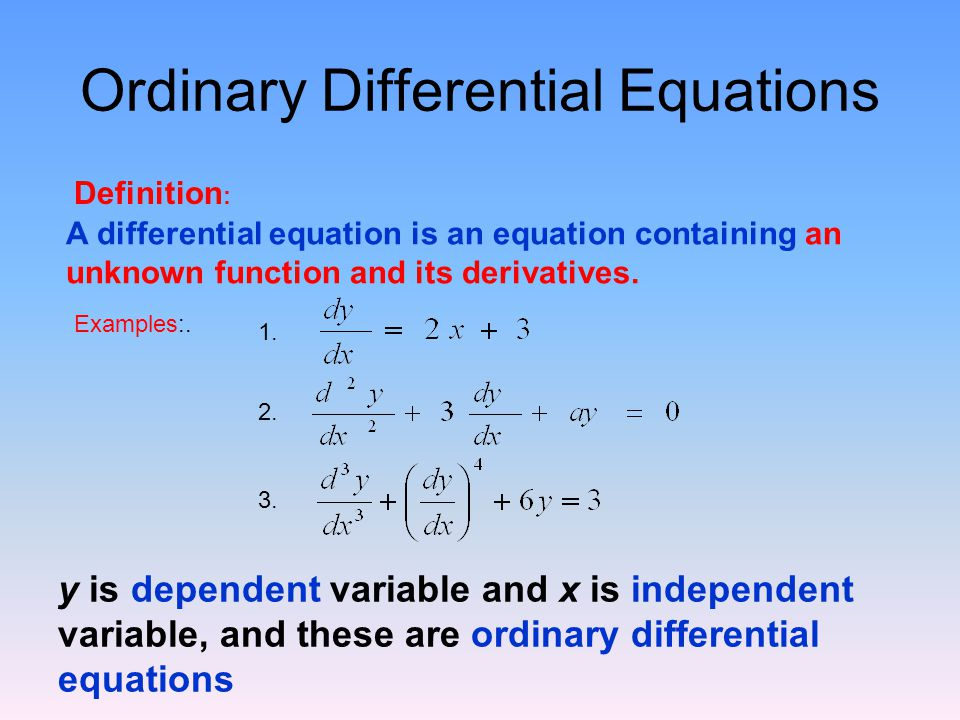Mathematics Quick revision Of Differential Equations For CBSE-NCERT

### Differential Equation=> In general, an equation involving derivative (derivatives) of the dependent variable with respect to independent variable (variables) is called a differential equation.

=> A differential equation involving derivatives of the dependent variable with respect to only one independent variable is called an ordinary differential equation, e.g.,

color{orange} {2 (d^y)/(dx^2) + ((dy)/(dx) )^3 = 0} is an ordinary differential equation.

### Order And Degree of a differential equation

Order of a differential equation :Degree of a differential equation :### General and Particular Solutions of a Differential Equation

color{red}{=>"The solution which contains arbitrary constants is called the general solution (primitive) of the differential equation."}

color{red}{=>" The solution free from arbitrary constants i.e., the solution obtained from the general solution"}
color{red}{" by giving particular values to the arbitrary constants is called a particular solution of the differential equation."}### Formation of differential equation### Solution of differential equation

(i) By variable separable method : separate the variable with dx and dy and solve the eqaution

(ii) Solution of homogeneous differential equation :### Solution of linear differential equation

(i) Write the given differential equation in the form (dy)/(dx) + Py = Q where where P, Q are constants or functions of x only.

(ii) Find the Integrating Factor (I.F) = color {red} {e^(int Pdx)}

(iii) Write the solution of the given differential equation as

color {red} {y (I.F) = ∫ (Q × I.F)dx + C}

\color{green} ✍️ In case, the first order linear differential equation is in the form (dx)/(dy) + P_1x =Q_1

=> where, P_1 and Q_1 are constants or functions of y only. Then I.F =e^( ∫ P_1 dy ) and the solution of the differential equation is given by

color {red} {x . (I.F) = ∫ (Q_1 × I.F ) dy + C}